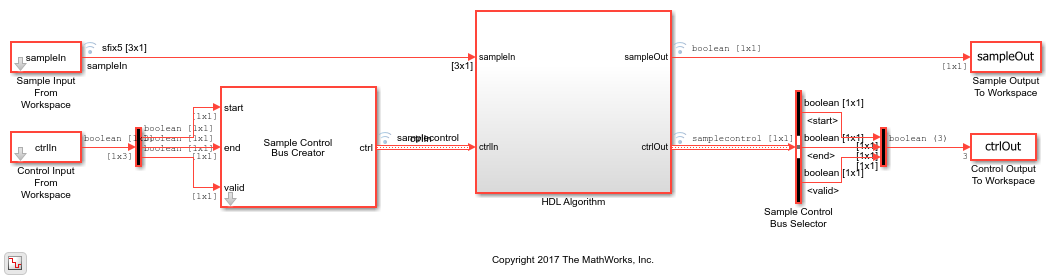# Convolutional Decode of Streaming Samples

This example shows how to use the LTE Convolutional Decoder block to decode data, and how to compare the hardware-friendly design with the results from LTE Toolbox™. The workflow follows these steps:

1. Generate LTE convolutionally encoded messages in MATLAB®, using LTE Toolbox.

2. Call Communications Toolbox™ functions to perform BPSK modulation, transmission through an AWGN channel, and BPSK demodulation. The result is soft-bit values that represent log-likelihood ratios (LLRs).

3. Quantize the soft bits according to the signal-to-noise ration (SNR).

4. Convert framed input data to a stream of samples and import the stream into Simulink®.

5. To decode the samples using a hardware-friendly architecture, execute the Simulink model, which contains the LTE Convolutional Decoder block.

6. Export the stream of decoded bits to the MATLAB workspace.

7. Convert the sample stream back to framed data, and compare the frames with the original input frames.

Calculate the channel SNR and create the modulator, channel, and demodulator System objects. `EbNo` is the ratio of energy per uncoded bit to noise spectral density, in dB. `EcNo` is the ratio of energy per channel bit to noise spectral density, in dB. The code rate of the convolutional encoder is 1/3. Therefore each transmitted bit contains 1/3 of a bit of information.

```EbNo = 10; EcNo = EbNo - 10*log10(3); modulator = comm.BPSKModulator; channel = comm.AWGNChannel('EbNo',EcNo); demodulator = comm.BPSKDemodulator('DecisionMethod','Log-likelihood ratio'); ```

Generate input data frames. Encode the data, modulate the message, and add channel effects to the resulting constellation. Demodulate the transmitted constellation and generate soft-bit values. For the hardware-friendly model, convert the soft bits into a fixed-point data type. The optimal soft-bit quantization step size is a function of the noise spectral density, `No`.

```rng(0); messageLength = 100; numframes = 2; numSoftBits = 5; txMessages = cell(1,numframes); rxSoftMessages = cell(1,numframes); No = 10^((-EcNo)/10); quantStepSize = sqrt(No/2^numSoftBits); for k = 1:numframes txMessages{k} = randi([0 1],messageLength,1,'int8'); txCodeword = lteConvolutionalEncode(txMessages{k}); modOut = modulator.step(txCodeword); chanOut = channel.step(modOut); demodOut = -demodulator.step(chanOut)/4; rxSoftMessagesDouble = demodOut./quantStepSize; rxSoftMessages{k} = fi(rxSoftMessagesDouble,1,numSoftBits,0); end ```

Serialize input data for the Simulink model. Leave enough time between frames so that each frame is fully decoded before the next one starts. The LTE Convolutional Decoder block takes (2 * |messageLength|) + 140 cycles to complete decoding of a frame.

The LTE Convolutional Decoder block expects the input data to contain the three encoded bits interleaved.

• Hardware-friendly input: `G0_1 G1_1 G2_1 G0_2 G1_2 G2_2 ... G0_n G1_n G2_n`

• LTE Toolbox input: `G0_1 G0_2 ... G0_n G1_1 G1_2 ... G1_n G2_1 G2_2 ... G2_n`

```idleCyclesBetweenSamples = 0; idleCyclesBetweenFrames = 2 * messageLength + 140; samplesizeIn = 3; interleaveSamples = true; [sampleIn,ctrlIn] = whdlFramesToSamples(rxSoftMessages,... idleCyclesBetweenSamples,... idleCyclesBetweenFrames,... samplesizeIn,... interleaveSamples); ```

Run the Simulink model. Because of the added idle cycles between frames, the streaming input variables include enough cycles for the model to complete decoding of both frames.

```sampletime= 1; simTime = size(ctrlIn,1); modelname = 'ltehdlConvolutionalDecoderModel'; open(modelname); sim(modelname); ```The Simulink model exports `sampleOut` and `ctrlOut` back to the MATLAB workspace. Deserialize the output samples, and compare to the decoded frame.

```rxMessages = whdlSamplesToFrames(sampleOut,ctrlOut); fprintf('\nLTE Convolutional Decoder\n'); for k = 1:numframes numBitsDiff = sum(double(txMessages{k})-double(rxMessages{k})); fprintf([' Frame %d: Behavioral and ' ... 'HDL simulation differ by %d bits\n'], k, numBitsDiff); end ```
```Maximum frame size computed to be 100 samples. LTE Convolutional Decoder Frame 1: Behavioral and HDL simulation differ by 0 bits Frame 2: Behavioral and HDL simulation differ by 0 bits ```